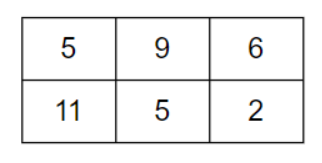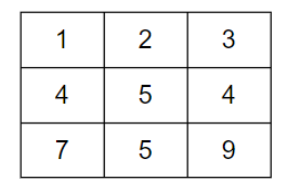0

# Minimum Path Sum

Difficulty: MEDIUM
Avg. time to solve
15 min
Success Rate
85%

Problem Statement
Suggest Edit

#### Find a path from top left i.e. (0, 0) to the bottom right i.e. ('N' - 1, 'M' - 1) which minimizes the sum of the cost of all the numbers along the path. You need to tell the minimum sum of that path.

##### Note:
``````You can only move down or right at any point in time.
``````
##### Input format :
``````The first line contains an integer 'T' denoting the number of test cases.

The first line of each test case contains two space-separated integers 'N' and ‘M’ representing the number of rows and number of columns in the grid, respectively.

Next 'N' lines will have 'M' space-separated integers, each line denotes cost values of that row.
``````
##### Output format:
``````For each test case, print the minimum sum of the path from top left to bottom right.
``````
##### Note:
``````You don't need to print anything, it has already been taken care of. Just implement the given function.
``````
##### Follow Up:
``````Can you solve this in O(1) space complexity?
``````
##### Constraints:
``````1 <= T <= 100
1 <= N, M <= 10^2
1 <= GRID[i][j] <= 10^5

Where 'GRID[i][j]' denotes the value of the cell in the matrix.

Time limit: 1 sec
``````
##### Sample Input 1:
``````2
2 3
5 9 6
11 5 2
1 1
5
``````
##### Sample Output 1:
``````21
5
``````
##### Explanation For Sample Output 1:
``````In test case 1, Consider a grid of 2*3:
````````````For this the grid the path with minimum value is (0,0) -> (0,1) -> (1,1) -> (1,2). And the sum along this path is 5 + 9 +5 + 2 = 21. So the ans is 21.

In test case 2, The given grid is:
````````````For this the grid the path with minimum value is (0,0) -> (0,1) -> (0,2) -> (1,2) -> (2,2).The sum along this path is 1 + 2 + 3 + 4 + 9 = 19.
``````
##### Sample Input 2:
``````2
2 2
5 6
1 2
3 3
1 2 3
4 5 4
7 5 9
``````
##### Sample Output 2:
``````8
19
``````
##### Explanation For Sample Output 2:
``````In test case 1, For this the grid the path with minimum value is (0,0) -> (1,0) -> (1,1). The sum along this path is 5 + 1 + 2 = 8.

In test case 2, The given grid is:
````````````For this the grid the path with minimum value is (0,0) -> (0,1) -> (0,2) -> (1,2) -> (2,2).The sum along this path is 1 + 2 + 3 + 4 + 9 = 19.
``````Console